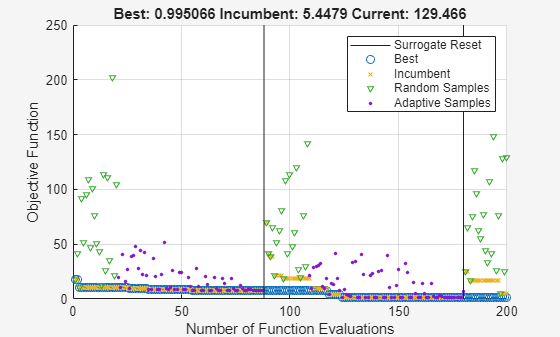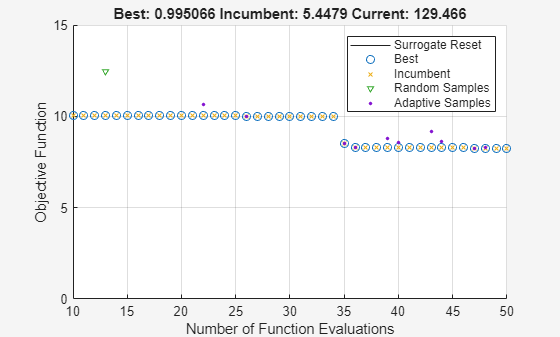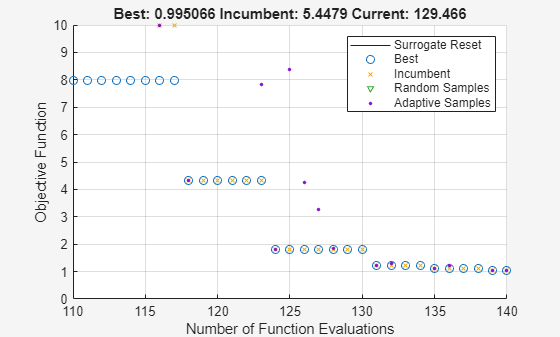## Interpret `surrogateoptplot`

The `surrogateoptplot` plot function provides a good deal of information about the surrogate optimization steps. For example, consider the plot of the steps `surrogateopt` takes on the built-in test function `rastriginsfcn`. This function has a global minimum value of 0 at the point [0,0]. By giving asymmetric bounds, you encourage `surrogateopt` to search away from the global minimum.

Create a surface plot of `rastriginsfcn`.

`ezsurf(@(x,y)rastriginsfcn([x(:),y(:)]));`Set asymmetric bounds of `[-3,-3]` and `[9,10]`. Set options to use the `surrogateoptplot` plot function, and then call `surrogateopt`.

```lb = [-3,-3]; ub = [9,10]; options = optimoptions('surrogateopt','PlotFcn','surrogateoptplot'); rng default [x,fval] = surrogateopt(@rastriginsfcn,lb,ub,options);``````Surrogateopt stopped because it exceeded the function evaluation limit set by 'options.MaxFunctionEvaluations'. ```

Begin interpreting the plot from its left side. For details of the algorithm steps, see Surrogate Optimization Algorithm.

• The first points are light blue triangles, indicating quasirandom samples of the function within the problem bounds. These points come from the Construct Surrogate phase.

• Next are black dots indicating the adaptive points, the points created in the Search for Minimum phase.

• The thick green line represents the best (lowest) objective function value found. Shortly after evaluation number 30, `surrogateopt` is stuck in a local minimum with an objective function value near 5. Zoom in to see this behavior more clearly.

```xlim([20 100]) ylim([0 10])```• Near evaluation number 115, a vertical line indicates a surrogate reset. At this point, the algorithm returns to the Construct Surrogate phase.

• The dark blue x points represent the incumbent, which is the best point found since the previous surrogate reset.

• Near evaluation number 150, the incumbent improves on the previous best point by attaining a value less than 1. After this evaluation number, the best point slowly drops in value to nearly zero. Zoom in to see this behavior more clearly.

```xlim([140 200]) ylim([0 6])```• After evaluation number 180 or so, most adaptive points are near the incumbent, indicating that the scale of the search shrinks.

• The optimization halts at evaluation number 200 because it is the default function evaluation limit for a 2-D problem.